# The SPP Procedure

### PROCESS Statement

• PROCESS name = (variables </pattern-options> )</process-options <distance-function-options>> ;

The PROCESS statement defines a point pattern for analysis. You must use a valid SAS variable name to define the process, and you can describe it by using variables that contain the x and y coordinates of the points within the point pattern. The variables must also be in the DATA= data set. You can specify only one PROCESS statement in PROC SPP.

The coordinates in spatial data can be spherical (represented as longitude and latitude) or projected (represented as Cartesian x and y coordinates). All the SAS/STAT procedures that analyze spatial data, including PROC SPP, assume that you are working with projected coordinates, for which Euclidean distance is appropriate. If your data consist of spherical coordinates, you are responsible for transforming the data to projected coordinates, such as by using PROC GPROJECT in SAS/GRAPH software. For more information about the spatial modeling issues that pertain to the use of geodetic versus simple Euclidean distance, see Banerjee (2005).

You can also specify pattern-options and process-options. The pattern-options are related to different attributes of the observed point pattern that is read from the DATA= data set. The process-options represent different analyses that are associated with a point pattern. These analyses are usually helpful in characterizing the underlying stochastic process that might have generated the point pattern. The PROCESS statement’s pattern-options are listed in Table 105.4. The PROCESS statement’s process-options are listed in Table 105.5.

Table 105.4: Point Pattern Definition Options

Option

Description

Specifies a rectangular study window

Specifies an EVENT variable that identifies individual point pattern events

Specifies the MARK variable for the point pattern

You can specify the following pattern-options, which enable you to describe various aspects of a point pattern data set:

AREA=(xmin-number, ymin-number, xmax-number, ymax-number)

specifies parameters that define the study area bounds for the spatial point pattern. This option describes is a key attribute that governs the intensity estimates that are obtained by different methods in PROC SPP. When you specify this option, you must identify all the following area specifications:

• xmin-number, the lower left limit for the x coordinate

• ymin-number, the lower left limit for the y coordinate

• xmax-number, the upper right limit for the x coordinate and

• ymax-number, the upper right limit for the y coordinate

If there are BY groups in the DATA= data set, then the explicit bounds remain the same across all BY groups. If you do not specify this option, then PROC SPP estimates a default area based on the Ripley-Rasson window estimator. For more information about the Ripley-Rasson window estimate, see the section Ripley-Rasson Window Estimator.

EVENT=variable-name

specifies an event variable that is associated with instances (points) in this point pattern. If your DATA= data set also contains information about covariates, use this option to identify the events in the point pattern.

MARK=variable-name

specifies a character or quantitative variable from the DATA= data set as a mark variable. Character variable marks are used for requesting distance function summary statistics across different variable values.

Table 105.5: PROCESS Statement Options

Option

Description

Computes the empty-space F function

Computes the G function

Computes the J function

Computes the K function to test for complete spatial randomness (CSR)

Obtains a nonparametric intensity estimate of the point pattern

Computes the L function

Specifies an output data set to store the simulated data sets in computation of distance functions

Computes the PCF function

Performs a quadrat based test for CSR

You can specify the following process-options to study the point pattern data set and the underlying spatial point process that is likely to have generated this pattern:

F<GRID(value-NX,value-NY)>

performs a test for complete spatial randomness that is based on the empty-space F function. For more information about the F function and related functions see the section Statistics Based on Second-Order Characteristics. You can specify the following suboption:

GRID(value-NX, value-NY)

specifies a reference grid for computing the empty-space F function, where value-NX represents the number of horizontal divisions and value-NY represents the number of vertical divisions. By default, the SPP procedure uses a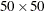grid.

G

performs a test for complete spatial randomness that is based on the nearest-neighbor G function.

J<GRID(value-NX, value-NY)>

performs a test for complete spatial randomness that is based on the J function. You can specify the following suboption:

GRID(value-NX, value-NY)

specifies a reference grid for computing the J function, where value-NX represents the number of horizontal divisions and value-NY represents the number of vertical divisions. By default, the SPP procedure uses agrid.

K

performs a test for complete spatial randomness that is based on the K function.

KERNEL<(kernel-suboptions)>

produces a nonparametric estimate of the first-order intensity, or a nonparametric smoothed estimate of a quantitative mark variable of the point pattern, depending on the kernel-suboptions. When you do not specify the kernel-suboptions, PROC SPP computes a nonparametric intensity estimate that is based on a default bandwidth and uses a Gaussian kernel. You can specify the following kernel-suboptions.

TYPE=EPANECHNIKOV | GAUSSIAN | QUARTIC | TRIANGULAR | UNIFORM

specifies the kernel type for obtaining the nonparametric estimate. For more information about the different kernel types that PROC SPP supports, see the section Nonparametric Intensity Estimation. By default, TYPE=GAUSSIAN.

B=value

specifies the value for the kernel bandwidth parameter. The bandwidth is a nonnegative number. By default, the SPP procedure uses a bandwidth of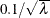, where
is the CSR average intensity of the point pattern (Illian et al. 2008, p. 236).

performs adaptive kernel estimation. Adaptive kernel estimation requires an initial bandwidth value to compute bandwidth estimates for each data point. If you specify a bandwidth in the B= kernel-suboption, then the SPP procedure uses this value as the initial bandwidth. Otherwise, it uses a default bandwidth value that is based on the suggestion by Illian et al. (2008, p.236). For more information about adaptive kernel estimation, see the section Nonparametric Intensity Estimation.

OUT=SAS-data-set

specifies the name of a SAS-data-set to contain the kernel based nonparametric estimates.

GRID(value-NX, value-NY)

specifies a reference grid for computing the kernel estimate, where value-NX represents the number of horizontal divisions and value-NY represents the number of vertical divisions. By default, the SPP procedure uses agrid.

L

performs a test for complete spatial randomness that is based on the L function.

OUTSIM=SAS-data-set

specifies the name of a SAS-data-set to contain the results of simulations in distance functions. This option is ignored unless one of the distance functions is specified in the PROCESS statement.

PCF<B=value>

performs a test for complete spatial randomness that is based on the pair correlation function (PCF) function. The pair correlation function is calculated only when you specify EDGECORR=ON in the PROC SPP statement. You can specify the following suboption:

B=value

specifies the bandwidth value to use in the kernel density estimation inside the pair correlation function. The value must be a nonnegative real number. Otherwise, it is assigned a default value of, whereis the CSR average intensity of the point pattern or of the current categorical mark type (Illian et al. 2008, p. 236).

performs a test for complete spatial randomness. You can specify value-NX and value-NY to provide a quadrat specification that includes the number of horizontal and vertical divisions. If you do not specify the number of horizontal and vertical divisions, PROC SPP computes a default quadrat of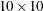. By default, the QUADRAT option displays only the Pearson chi-square test for CSR. If you also specify the DETAILS suboption, then PROC SPP displays the quadrat count in addition to the Pearson residual information.

When you specify an F, G, J, K, L, or PCF process-option (shown in Table 105.5), you can also specify the following distance-function-options.

Table 105.6: Distance Function Options

Option

Description

Requests categorical mark typewise calculation of distance functions

Requests cross-type distance function analysis that is based on the categorical mark that is specified in the MARK= option

Specifies the ending distance for distance functions

Specifies the starting distance for distance functions

Specifies the number of distances to use for different distance functions

Specifies the number of simulations to compute the CSR envelope

Specifies the block size for calculation of confidence intervals for distance functions

BYTYPE(ALL|value-list)

requests distance function calculation by values of the mark variable. This option produces individual distance function calculations for each mark type. You can specify the following options:

ALL

requests distance function calculation for all available character mark variable values in the DATA= data set.

value-list

requests distance function calculation for certain formatted mark variable values, which you specify as quoted strings in the value-list.

CROSS=TYPES(value-list1<,value-list2>)

requests cross-type distance function analysis between different mark values. For cross-type analysis, you must specify a mark variable in the point pattern definition by using the MARK= pattern-option. The CROSS= option applies only to any requested distance functions K, L, G, J, or PCF. You must specify the TYPES suboption as follows:

TYPES(value-list1<,value-list2>)

requests cross-type analysis only among types that are specified in value-list1 and an optional value-list2. If you specify only value-list1, then PROC SPP performs cross-type analysis within all the types that are specified in value-list1. If you also specify the additional value-list2, PROC SPP performs cross-type analysis across both lists. For value-list1 and value-list2, specify quoted strings that correspond to values of the variable that is specified in the MARK=pattern-option.

MAXDIST=value | MAX | CUT

specifies the option to be used for computing the maximum distance for different distance functions. You can specify the following options:

value

specifies a value for the maximum distance for performing distance function calculations. The value must be positive and larger than the value of the MINDIST=value option. You can specify any positive value for the maximum distance. However, values that are too large might produce artifacts that do not reflect the true underlying process.

MAX

uses the maximum possible distance, based on the suggestion by Baddeley and Turner (2013). The maximum possible distance is calculated as follows:

• For the K and L functions, the maximum possible distance is calculated as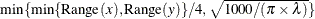whereis the intensity of the point pattern in the study area and the ranges of x and y are computed over the minimum bounding rectangular window of the study area.

• For the PCF functions, the maximum possible distance is calculated as in the case of K and L functions except that the ranges of x and y are computed over a block division of the study area and thecorresponds to the intensity in a block division. The computed maximum distance for the PCF distance is the minimum of the maximum distance computed over all the block divisions in the study area.

• For the F and G functions, the maximum possible distance is calculated as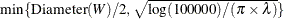whereis the intensity of the point pattern in the study area and W is the minimum bounding rectangular window of the study area.

• For the J function, the maximum possible distance is calculated as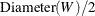.

CUT

uses the maximum distance at certain cutoff values that are recommended by Baddeley (2014). The cutoff values are as follows:

• for the F and G functions, the distance at which the F or G value reaches 0.9

• for the J function, the distance at which the F or G value in the calculation of the J function reaches 0.9

• for the PCF function, the distance that corresponds to the MAX option that is applied to individual subdivisions of the study area for computing the confidence interval of the PCF statistic

• for the K and L functions, the distance that corresponds to theMAX option that is applied to the entire study area

By default, PROC SPP uses the value of MAXDIST is CUT.

MINDIST=value

specifies a positive number for the minimum distance (or starting) distance for all distance function calculations. The value of this option cannot be more than the value of MAXDIST= option.

NDIST=value

specifies the number of distance bins with which to compute all the specified distance functions. This is a global option that applies to all specified distance functions. When you specify a value for this option, the SPP procedure uses this value instead of others for distance function calculations.

NSIM=value

specifies a positive integer for the number of simulations to be used to compute envelopes for the CSR tests in all distance functions. When you specify this option, it applies to all specified distance functions.

BLOCKS(NX, NY)

specifies the block size that is required for calculating the confidence intervals of distance functions, where NX specifies the number of horizontal blocks and NY specifies the number of vertical blocks. The block size should be neither too small nor too large for this option to behave reasonably. For more information about estimating the confidence intervals for distance functions see the section Confidence Intervals for Summary Statistics. The default block size is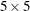.